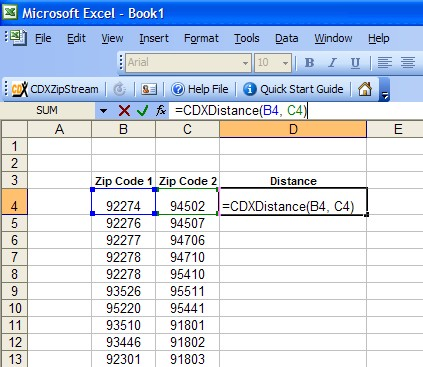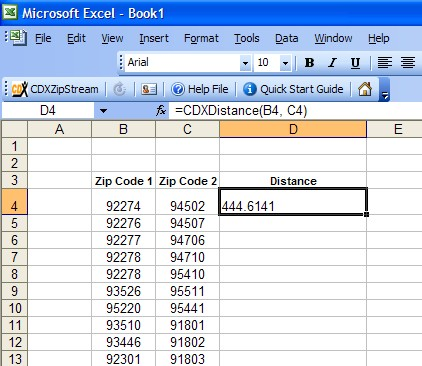CDXDistance Function Previous  Top  Next

CDXDistance is a custom function available in CDXZipStream. The CDXDistance function calculates straight line ("as the crow flies") distances between zip codes. If you have purchased the Canadian postal code database, you can also use it to calculate distances between postal codes. To calculate driving distances and times, please use the CDXRouteMP function.

In cases where staight line distances between lists of zip codes must be calculated, input the CDXDistance custom function formula as shown below:In this case the first pair of zip codes are in cells B4 and C4, and the custom function formula is:

=CDXDistance(B4, C4)

which results in a calculated distance of 444.6141 miles:To apply this function to the remaining zip codes, copy and paste the custom function formula. In this case, we copy cell D4 and paste it to the range D5 through D13:This cut and paste technique will also work if the zip codes are listed in rows instead of columns as shown here.

The calculated distance is automatically provided in miles, but you can specify kilometers or nautical miles using the following formulas:

For kilometers: = CDXDistance(B4, C4, "KM")
For nautical miles: = CDXDistance(B4, C4, "NM")